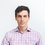# Confidence intervals in estimation

The $\pm$ in polling is a measure of the best estimate the pollsters have for the error in their estimation of population preference. If they were to repeat the poll on infinitely many subsets of $N$ people out of the population $N_{tot}$, what kind of spread do they expect to find around the true result, $\langle A \rangle = Np_A$? I.e. what is the variance in the preference of the sample populations?

If $\hat{A}$ represents the number of people with opinion $\mathbb{A}$ in a given sample, the sample standard deviation is given by

$\sqrt{\langle\hat{A}^2\rangle - \langle\hat{A}\rangle^2}$

This sample standard deviation is the intrinsic variation one expects to find in repeatedly estimating the sample mean due to undersampling the population: If the full population has the true frequency $p_A$, how much can we expect our sample frequency $\hat{p}_A$ to deviate from $p_A$?

Clearly, the probability of obtaining $\hat{p}_A$ as our sample frequency is greatest for a true frequency centered at $\hat{p}_A$ itself. However, we can expect to find a sample frequency $\hat{p}_A$ for a variety of true frequencies. To circumvent the fact that we are completely ignorant to the value of the true frequency, we can try to establish an interval within which we are confident the true result lies.

We can find the endpoints of this interval by asking what is the largest value of the true population frequency, $p^H_A < \hat{p}_A$, for which $\hat{p}_A$ would be an unlikely result? Likewise, what is the smallest value of the true frequency, $p^L_A$, for which $\hat{p}_A$ would be unlikely? By doing so, we establish lower and upper bounds on the interval.

For instance, if we'd like a 95% confidence interval, we search for the value of $p^H_A$ such that $p(\hat{p}_A \mid p_A = p^H_A) < 2.5\%$, and $p^L_A$ such that $p(\hat{p}_A \mid p_A = p^L_A) < 2.5\%$.

This is better expressed visually. We see that if we measure $\hat{p}_A$ (black arrow in figure), it could conceivably be a result of sampling the distribution on the left, in which case $\hat{p}_A$ is an overestimate, or of sampling the distribution on the right, in which case it is an underestimate.As shown in the cartoon plot, these confidence intervals are, in general, asymmetric as the true frequency moves away from $p_A = \frac12$. Contrast this with typical reporting practices which assert errors of the form $\pm x$, suggesting that the uncertainty is the same in both directions.

As an extreme counterexample, consider a case where a sample of 50 people suggests a frequency of 0.02. This could arise when testing for a rare medical condition, or the distribution of adverbs in a sample of Victorian literature. Although the error can be quite high, above 2%, it is clear that we cannot possibly have something like $2\%\pm 3\%$, as a $101\%$ frequency is nonsensical. In these cases, the standard deviation is not a good measure of uncertainty.

However, for sufficiently large values of $N$, and values of $p_A$ not too close to zero or one, it is not a terrible simplification to approximate the uncertainty about the sample frequency as a symmetric confidence interval. Under this approximation, we can take the standard deviation as a symmetric measure of uncertainty, and assume the true frequency to be normally distributed about the sample frequency.As it turns out, political polls tend to have $p$ close to 0.5 (why is this?), so the approximation is valid.

The question is how to calculate these quantities. Next, we will motivate a simple derivation of the error in public polls by analogy with a concept from statistical mechanics.Note by Josh Silverman
7 years, 3 months ago

This discussion board is a place to discuss our Daily Challenges and the math and science related to those challenges. Explanations are more than just a solution — they should explain the steps and thinking strategies that you used to obtain the solution. Comments should further the discussion of math and science.

When posting on Brilliant:

• Use the emojis to react to an explanation, whether you're congratulating a job well done , or just really confused .
• Ask specific questions about the challenge or the steps in somebody's explanation. Well-posed questions can add a lot to the discussion, but posting "I don't understand!" doesn't help anyone.
• Try to contribute something new to the discussion, whether it is an extension, generalization or other idea related to the challenge.

MarkdownAppears as
*italics* or _italics_ italics
**bold** or __bold__ bold
- bulleted- list
• bulleted
• list
1. numbered2. list
1. numbered
2. list
Note: you must add a full line of space before and after lists for them to show up correctly
paragraph 1paragraph 2

paragraph 1

paragraph 2

[example link](https://brilliant.org)example link
> This is a quote
This is a quote
    # I indented these lines
# 4 spaces, and now they show
# up as a code block.

print "hello world"
# I indented these lines
# 4 spaces, and now they show
# up as a code block.

print "hello world"
MathAppears as
Remember to wrap math in $$ ... $$ or $ ... $ to ensure proper formatting.
2 \times 3 $2 \times 3$
2^{34} $2^{34}$
a_{i-1} $a_{i-1}$
\frac{2}{3} $\frac{2}{3}$
\sqrt{2} $\sqrt{2}$
\sum_{i=1}^3 $\sum_{i=1}^3$
\sin \theta $\sin \theta$
\boxed{123} $\boxed{123}$